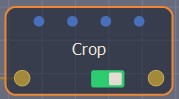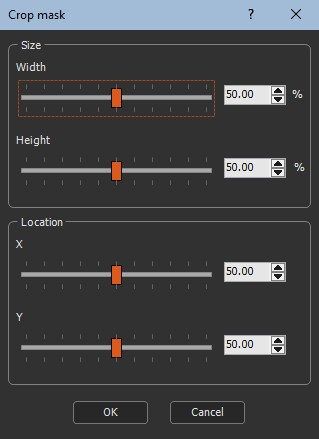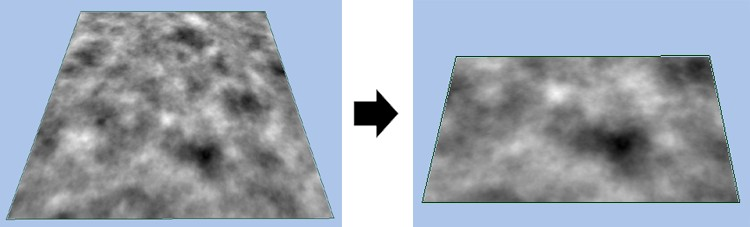This node retrieves an input mask and Crops it in order to output only a part of the mask.

To add a Crop node, right-click in the Graph Editor and select Create Node  > Mask transformation  > Crop.Double click on the node to open its parameters:Use the sliders to adjust the Crop values:

• Width: New width as a percentage of the initial width (between a minimum percentage and 100); the minimum percentage is a new width of 16 vertices*.
• Height: New height as a percentage of the initial width (between a minimum percentage and 100); the minimum percentage is a new height of 16 vertices*.
• X: Location of the X-axis Crop from 0 (left) to 100 (right).
• Y: Location of the Y-axis Crop from 0 (top) to 100 (bottom).

The default values are width: 50; height: 50; X: 50; Y: 50, or a quarter of the mask, and centered.

*The size in meters depends on your quad size. If the quad size = 1, the minimum size allowed is 15 meters, if the quad size = 0.5, the minimum size allowed is 7.5m, etc.

For example, setting the parameters to width: 60; height: 40; X: 60; and Y: 90 gives the following results:Parameters

Parameter Use
Width New width as a percentage of the initial width (between a minimum percentage and 100); the minimum percentage is a new width of 16 vertices
Height New height as a percentage of the initial width (between a minimum percentage and 100); the minimum percentage is a new height of 16 vertices
X Location of the X-axis Crop from 0 (left) to 100 (right)
Y Location of the Y-axis Crop from 0 (top) to 100 (bottom)